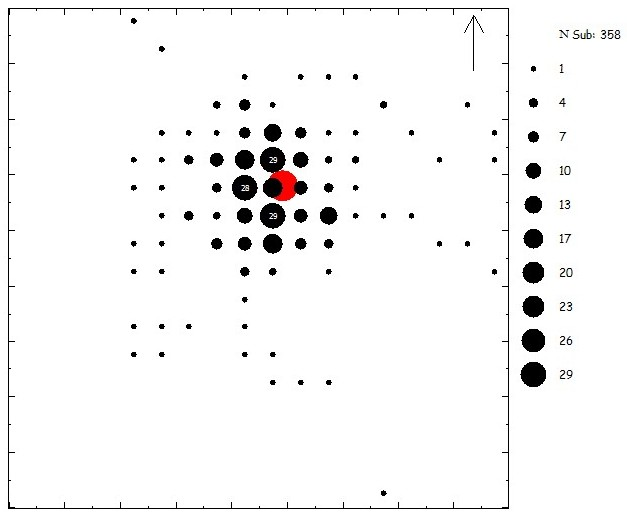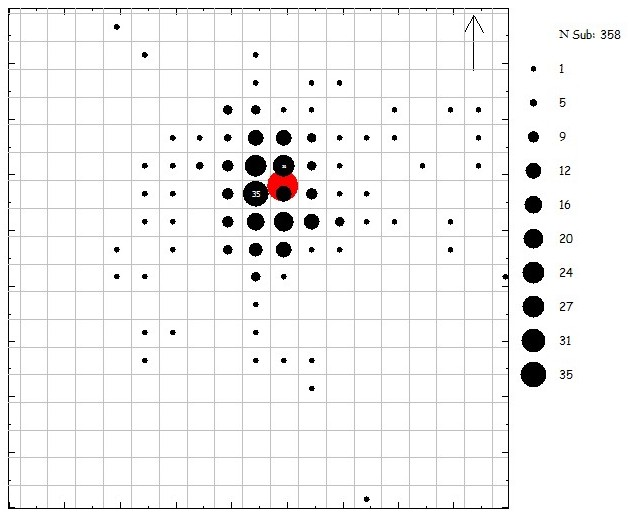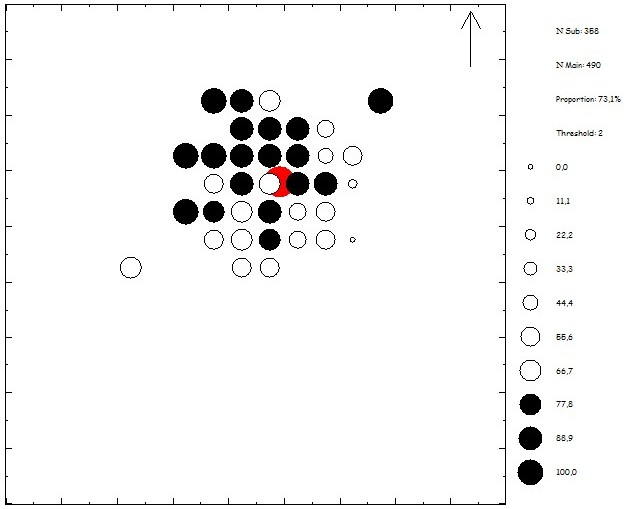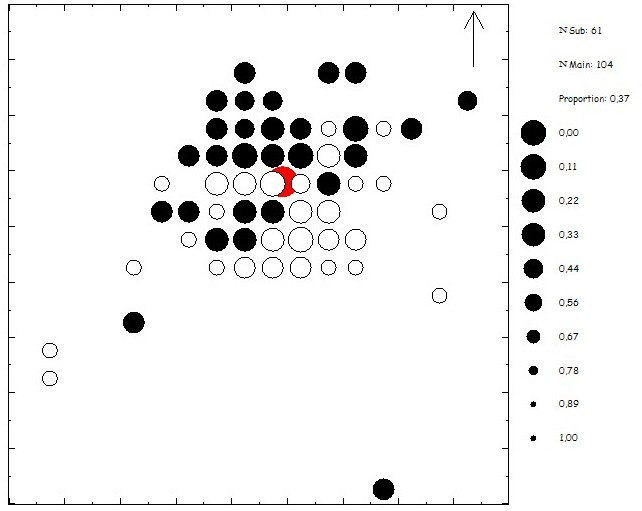## Density analysis

For a density map the artefact frequencies are counted per grid cell. In each cell the frequency is represented by a circle. The relative number of artefacts in a cell determines how large the circle will be. There are three different ways to calculate the size of circles: peripheral, linear and central. The peripheral option stresses the lower frequencies, and the central option stresses the higher frequencies. However, the appearance of a circle is determined by its surface area, and not by its radius; therefore the peripheral option is in fact linear.

ANALITHIC offers the possiblity to optimize the position of the grid, for example by calculating the richest cell.

The frequencies for each cell can be shown by creating a frequency map. Frequencies for the higher cell values can also be shown in the density map it self.

By comparing two selections a proportion map or an exact probability map is created.

#### Some examples:Pincevent Unit T112: density map of the tools. The higher cell values are shown inside the rounds. The size is calculated by using the peripheral option, stressing the lower values.Pincevent Unit T112: this figure also diplays a density map with tools, but now the grid prosition is optimized by using the richest cell option. The richest cell now contains 35, instead of 29 artefacts.Pincevent unit T112: proportion map of tools compared to all artefacts. Black rounds have proportionally more tools. The threshold value is set to 2; cells with frequencies of 2 or less or not shown on this map.Pincevent unit T112: exact probability map of burins and burin spalls. For each cell the p-value is calculated with the binomial function. Note that the lower the p-value, the bigger the circle.